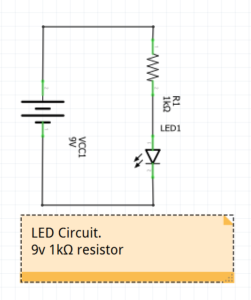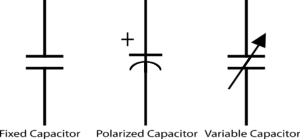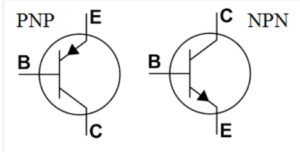Resistors

https://en.wikipedia.org/wiki/ResistorResistors are measured in Ohms Ω. They are used to limit the current that flows through the circuit. Resistors mostly come in a fixed numeric.

1, 1.5, 2, 2.2, 3.3, 4.7 and then multiples of that. e.g. 1.5kΩ, 2.2MΩ.

The most basic algorithm used with resistors is Ohms law.

v = i * r

Voltage = Current * Resistance.

For example if you have a 9v battery and a 1kΩ (1000 ohms) resistor. The current drawn will be:

i = v / r = 9 / 1000 = 9mA (9 milliamps).

If this was in  a simple circuit for lighting an LED it would limit the current and hence the brightness of the LED.Capacitors

https://en.wikipedia.org/wiki/Capacitor

Capacitors are measured in Farads (Transistors

https://en.wikipedia.org/wiki/TransistorThe two basic types of transistors we are covering are NPN and PNP.

Transistor act like switches. If there is a current applied to the base it turns the switch on or off depending on the type.

Diodes

https://en.wikipedia.org/wiki/DiodeDiodes allow current to pass in only one direction. This means that if they are connected the wrong way around, the the circuit will not work as you expect.

LEDshttps://en.wikipedia.org/wiki/Light-emitting_diode

Light Emitting Diodes. The symbol for an LED is the symbol for a diode with an added arrow.

Like a diode, current can only pass in one direction through an LED. Unlike a diode, the LED emits light when current passes through it.